## C语言编写管道代码

int pipe（int fd）;

fd:文件描述符数组，其中fd表示读端，fd表示写端。

``````#include <stdio.h>
#include <unistd.h>
#include <sys/types.h>
#include <sys/stat.h>
#include <fcntl.h>
#include <stdlib.h>
#include <errno.h>
#include <string.h>
#include <dirent.h>
#include <signal.h>
#include <sys/wait.h>
#include <sys/time.h>
#define ERR_EXIT(m)				\
do						     \
{						     \
perror(m);				\
exit(EXIT_FAILURE);		\
}while(0)

int main()
{
int pipefd;
if(pipe(pipefd) == -1)
{
ERR_EXIT("pipe error");
}
pid_t pid;
pid = fork();
if(pid == 0)
{
close(pipefd);
write(pipefd,"hello",5);
close(pipefd);
exit(EXIT_SUCCESS);
}
close(pipefd);
char buf = {0};
printf("buf = %s\n",buf);
return 0;
}``````

``````	int pipefd;
if(pipe(pipefd) == -1)
{
ERR_EXIT("pipe error");
}
``````

``````	pid_t pid;
pid = fork();
if(pid == 0)
{
close(pipefd);
write(pipefd,"hello",5);
close(pipefd);
exit(EXIT_SUCCESS);
}
``````

close(pipefd);这行代码的意思是，关闭读端。因为子进程是要写字符串在管道中，所以读端就没什么用了。关闭了。

write(pipefd,"hello",5)；这行代码的意思就是向管道中写hello这个字符串。

``````	close(pipefd);
char buf = {0};
printf("buf = %s\n",buf);
return 0;``````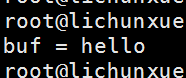``````#include <stdio.h>
#include <unistd.h>
#include <sys/types.h>
#include <sys/stat.h>
#include <fcntl.h>
#include <stdlib.h>
#include <errno.h>
#include <string.h>
#include <dirent.h>
#include <signal.h>
#include <sys/wait.h>
#include <sys/time.h>
#define ERR_EXIT(m)				\
do						     \
{						     \
perror(m);				\
exit(EXIT_FAILURE);		\
}while(0)

int main()
{
int pipefd;
if(pipe(pipefd) == -1)
{
ERR_EXIT("pipe error");
}
pid_t pid;
pid = fork();
if(pid == 0)
{
dup2(pipefd,STDOUT_FILENO);
close(pipefd);
close(pipefd);
execlp("ls","ls",NULL);
fprintf(stderr,"error execor execte ls\n");
exit(EXIT_FAILURE);
}
dup2(pipefd,STDIN_FILENO);
close(pipefd);
close(pipefd);
execlp("wc","wc","-w",NULL);
fprintf(stderr,"error execute wc\n");
return 0;
}
``````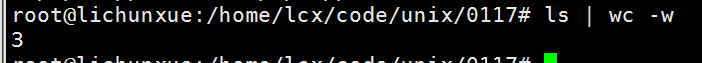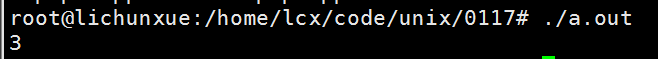·重复部分不做解释，只简单说明一下：

``````	if(pid == 0)
{
dup2(pipefd,STDOUT_FILENO);
close(pipefd);
close(pipefd);
execlp("ls","ls",NULL);
fprintf(stderr,"error execor execte ls\n");
exit(EXIT_FAILURE);
}
``````

dup2(pipefd,STDOUT_FILENO);这行代码的意思是，把文件输出描述符定义到写端。

close(pipefd);
close(pipefd);

execlp("ls","ls",NULL);
fprintf(stderr,"error execor execte ls\n");

dup2(pipefd,STDIN_FILENO);

execlp("wc","wc","-w",NULL);

``````#include <stdio.h>
#include <unistd.h>
#include <sys/types.h>
#include <sys/stat.h>
#include <fcntl.h>
#include <stdlib.h>
#include <errno.h>
#include <string.h>
#include <dirent.h>
#include <signal.h>
#include <sys/wait.h>
#include <sys/time.h>
#define ERR_EXIT(m)				\
do						     \
{						     \
perror(m);				\
exit(EXIT_FAILURE);		\
}while(0)

int main()
{
close(0);
open("pip.cpp",O_RDONLY);
close(1);
open("01pipe.cpp",O_WRONLY | O_CREAT | O_TRUNC,0644);
execlp("cat","cat",NULL);
return 0;
}
``````

``````#include <stdio.h>
#include <unistd.h>
#include <sys/types.h>
#include <sys/stat.h>
#include <fcntl.h>
#include <stdlib.h>
#include <errno.h>
#include <string.h>
#include <dirent.h>
#include <signal.h>
#include <sys/wait.h>
#include <sys/time.h>
#define ERR_EXIT(m)				\
do						     \
{						     \
perror(m);				\
exit(EXIT_FAILURE);		\
}while(0)

int main()
{
close(0);
open("04pipe.cpp",O_RDONLY);
execlp("cat","cat",NULL);
return 0;
}
``````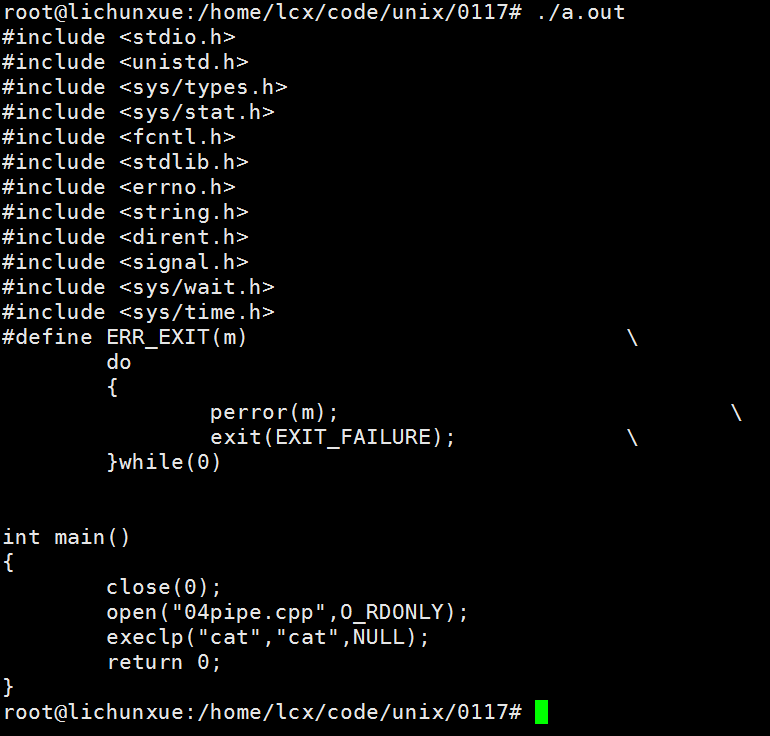https://blog.csdn.net/m0_38036750/article/details/85066109

``````mkfifo filename
``````

int mkfifo(const char *filename,mode_t mode);

``````#include <stdio.h>
#include <unistd.h>
#include <sys/types.h>
#include <sys/stat.h>
#include <fcntl.h>
#include <stdlib.h>
#include <errno.h>
#include <string.h>
#include <dirent.h>
#include <signal.h>
#include <sys/wait.h>
#include <sys/time.h>
#define ERR_EXIT(m)				\
do						     \
{						     \
perror(m);				\
exit(EXIT_FAILURE);		\
}while(0)

int main()
{
mkfifo("p2",0644);
return 0;
}
``````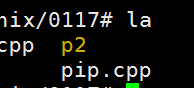p2是一个管道文件，这个和mkfifo filename相似。

FIFO（命名管道）与pipe（匿名管道）之间唯一的区别在他们创建与打开的方式不同，一但这些工作完成之后，他们具有相同的语义。

O_NONBLOCK disable：阻塞直到有相应进程为写而打开该FIFO

O_NONBLOCK enable：立刻返回成功

O_NONBLOCK disable：阻塞直到有相应进程为读而打开该FIFO

O_NONBLOCK enable：立刻返回失败，错误码为ENXIO